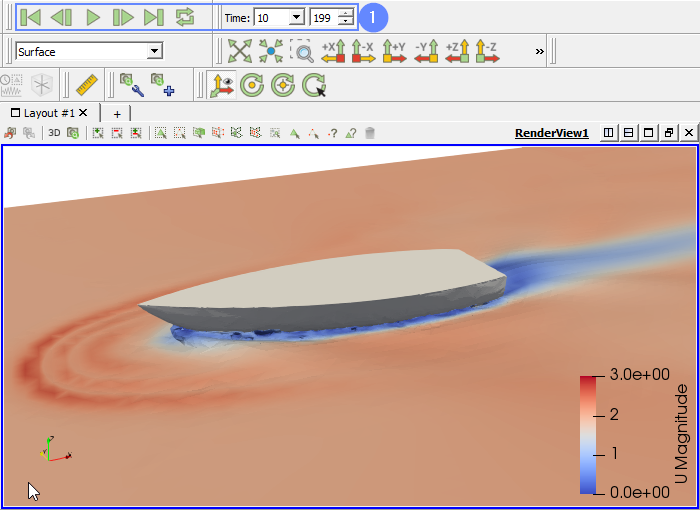Back to all tutorials

# Ship Hull - CFD Simulation SimFlow Tutorial

## 1. Introduction

In this tutorial, you’ll discover how to simulate mesh deformation in SimFlow. An instance of this is the transformation of a 6DOF mesh, which results from the forces acting on a ship’s hull while it sails. The simulation considers a two-phase flow consisting of water and air, as well as Dynamic Mesh functionality with applied constraints.

SimFlow is a general purpose CFD Software

## 3. Create Case

Open SimFlow and create a new case named Boat

1. Go to panel
2. Provide name Boat
3. Click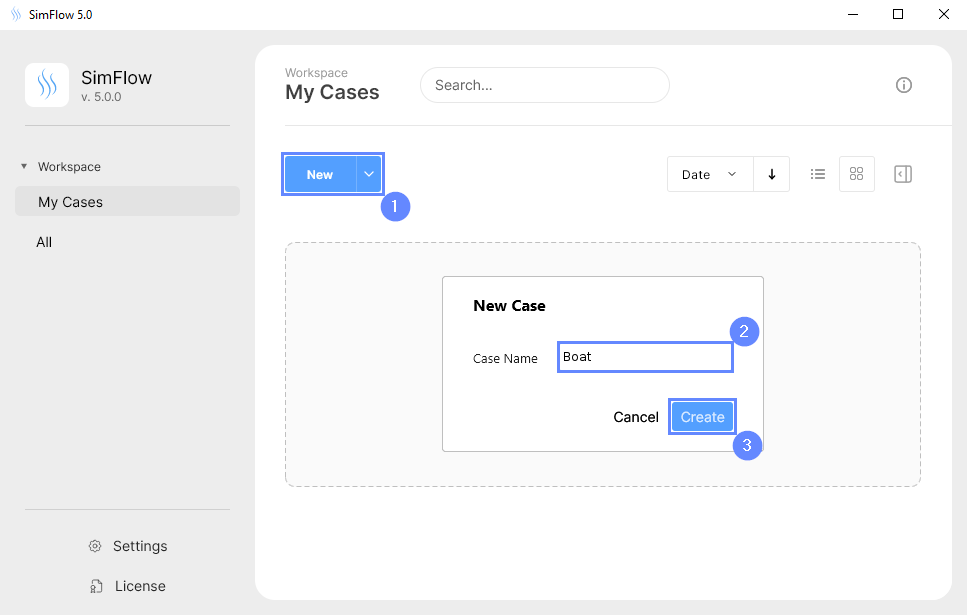## 4. Import Geometry - Boat

Firstly we need to Download GeometryBoat. The geometry will be imported in the same units as it was exported to the STEP format.

1. Click
2. Select geometry file Boat.step
3. Click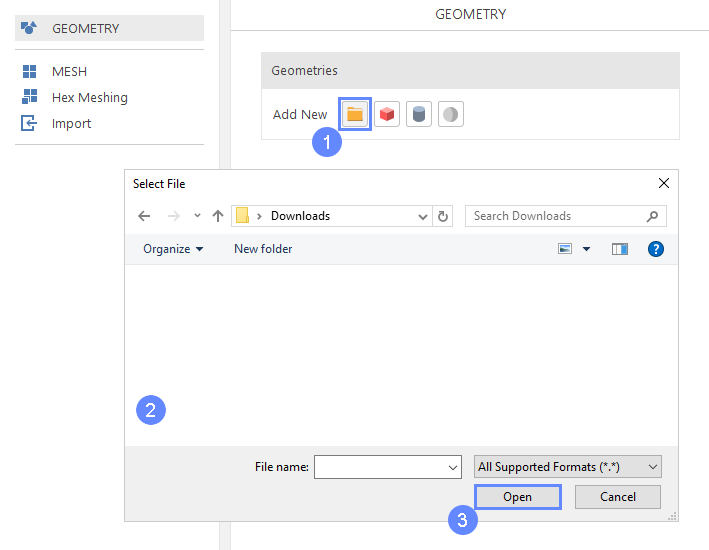## 5. Preview Geometry - Boat

After importing geometry, it will appear in the 3D window.

1. Click to zoom in on the geometry.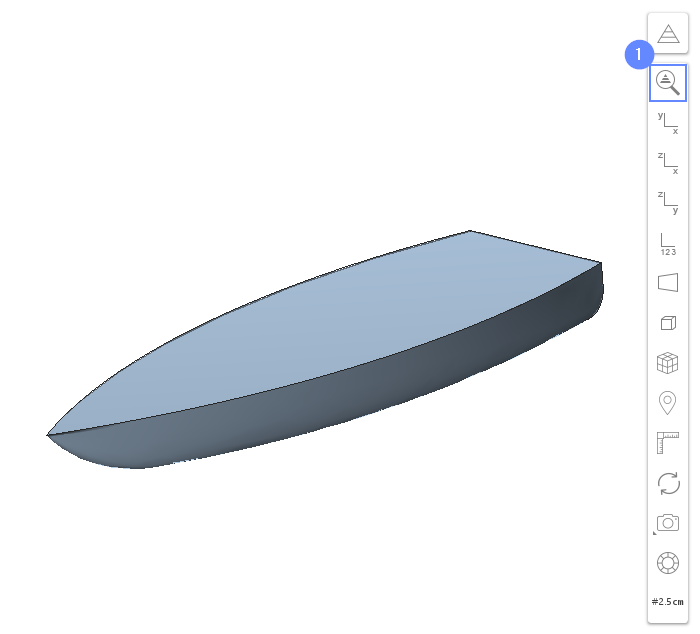## 6. Rotate Geometry - Boat (I)

We will rotate the model about X axis at 90 deg to orient the Z axis vertically.

1. Expand the list for Boat
2. Select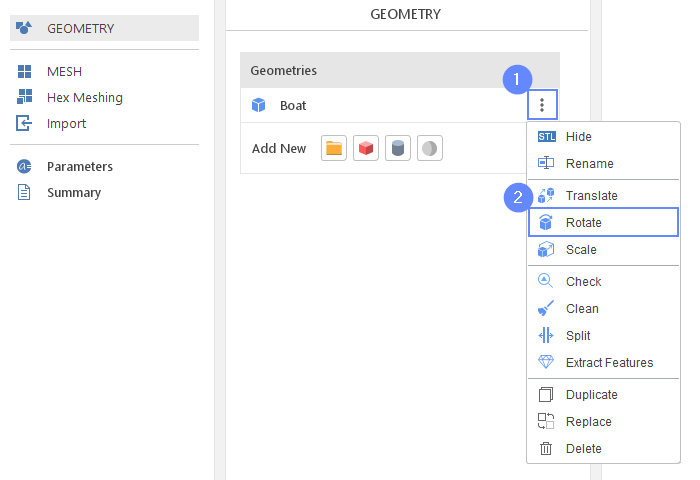## 7. Rotate Geometry - Boat (II)

1. Set the following parameters accordingly
Axis $${\sf [-]}$$100
Angle $${\sf [deg]}$$90
2. Click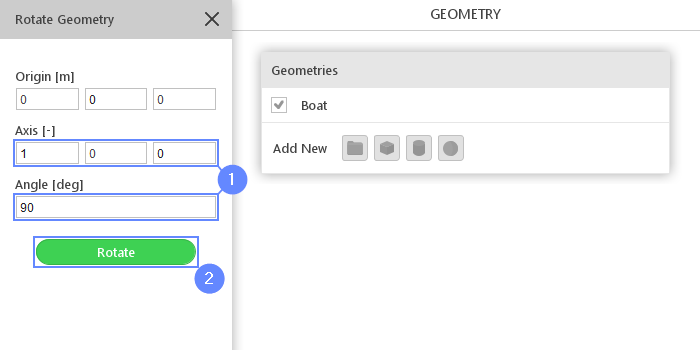## 8. Create Geometry - Water

Additionally, to the hull geometry, we will create a box that will be used to indicate the initial water location.

1. Select
2. Change geometry name from box_1 to water
double click to edit name and press Enter to confirm
3. Set the origin and box dimensions:
Origin $${\sf [m]}$$-11-6-3
Dimensions $${\sf [m]}$$22122.85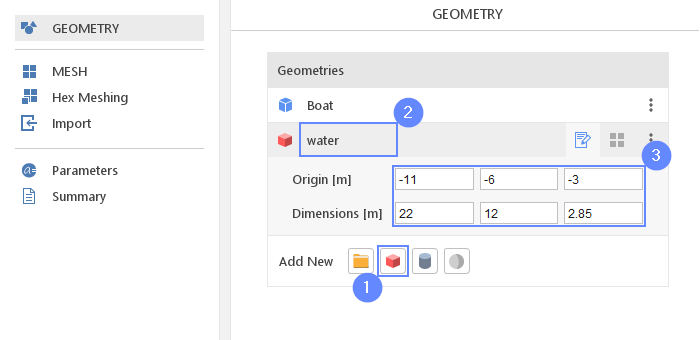## 9. Create Geometry - Refinement

We need to refine the mesh near the water surface to increase the accuracy of the results. For this purpose, we will create also a refinement box.

1. Select
2. Change geometry name from box_1 to refinement
3. Set the origin and box dimensions
Origin $${\sf [m]}$$-11-6-0.35
Dimensions $${\sf [m]}$$22120.4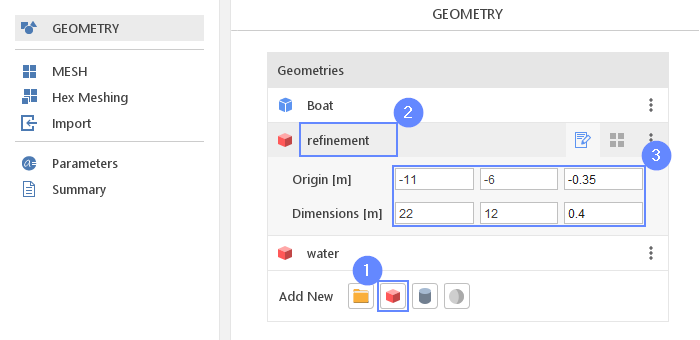## 10. Meshing Parameters - Boat

In order to create the mesh, we need to specify geometries options for the meshing process.

1. Go to Hex Meshing panel
2. Select Boat geometry
3. Enable
4. Enable
5. Set Refinement to Min 4 Max 4
6. Set No. of Layers to 4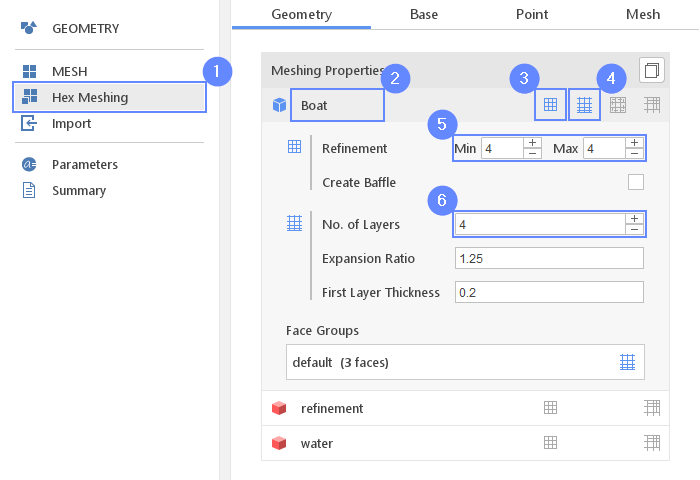## 11. Meshing Parameters - Refinement

1. Click on the refinement geometry
2. Enable
3. Set the refinement Level to 2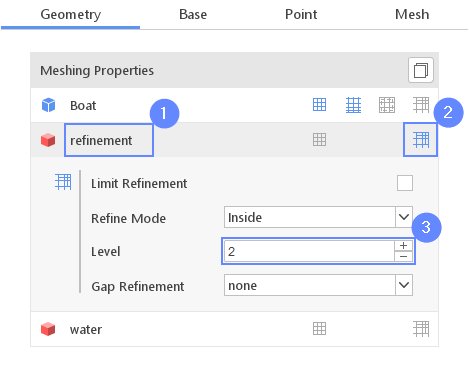## 12. Base Mesh - Domain

Now, we will define the base mesh. The box geometry determines the background mesh domain. The model is symmetrical with respect to the XZ plane and we can take advantage of this fact. Using box dimensions we will choose only half of the required domain.

1. Go to Base tab
2. Set the size of the base mesh:
Min $${\sf [m]}$$-100-2
Max $${\sf [m]}$$1051
3. Define the number of divisions
Division551413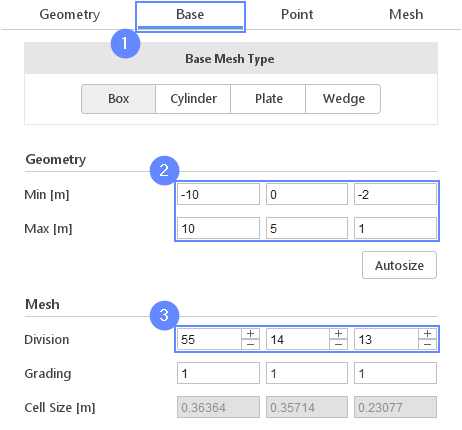## 13. Base Mesh - Boundaries

We need to assign individual names to each side of the base mesh. This will allow us to apply different conditions to each side.

1. Define boundary names and types accordingly
X- inlet patch
X+ outlet patch
Y- symmetry symmetry
Y+ symmetry symmetry
Z- bottom wall
Z+ top patch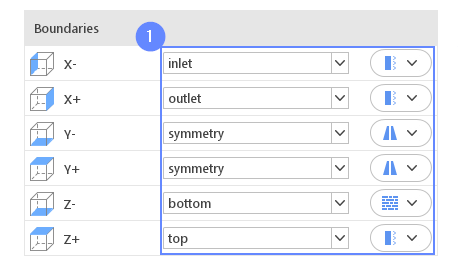## 14. Material Point

Material Point tells the meshing algorithm on which side of the geometry the mesh is to be retained. Since we are considering fluid outside the boat, we need to place the material point outside the geometry.

1. Go to Point tab
2. Specify location inside base mesh, but outside boat geometry
Material Point020
 You can specify the point location from the 3D view. Hold the Ctrl key and drag the arrows to the destination.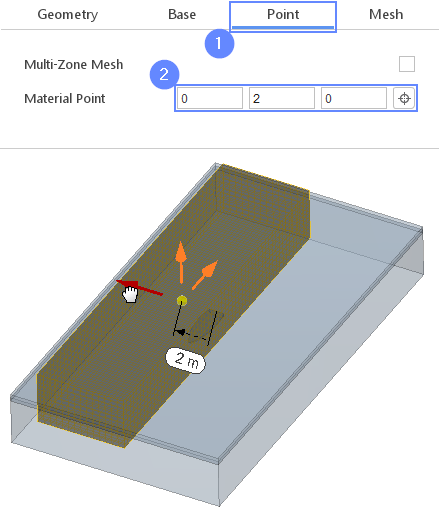## 15. Meshing Settings

We can define the count of the buffer cells between refinement levels.

This parameter determines the width of the transition zone between refined and background mesh. Lowering this parameter will reduce the overall cells count.

1. Go to Mesh tab
2. Go to Settings
3. Set Cells Between Levels to 3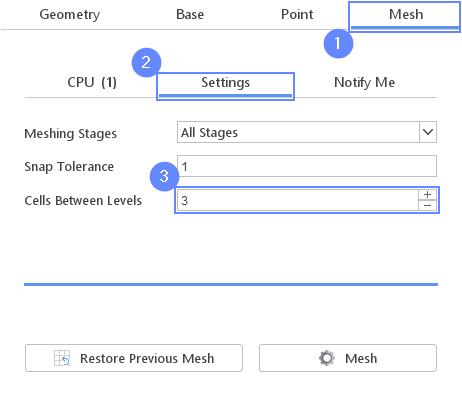## 16. Start Meshing

In this step we will initiate the meshing process.

In the meshing panel you may indicate how many CPUs would you like to use for this process. Please note that if you are using SimFlow free version you may only use serial meshing, and you may not create meshes larger than 200'000 nodes.

If you would like to test full version Request 30-day Trial

1. Go to CPU tab
2. Press the button to start meshing process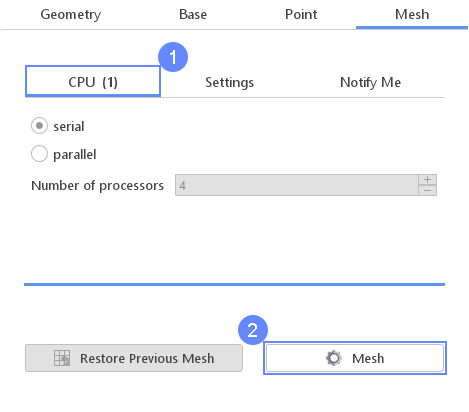## 17. Mesh

After the meshing process is finished, the mesh will appear in the graphics window.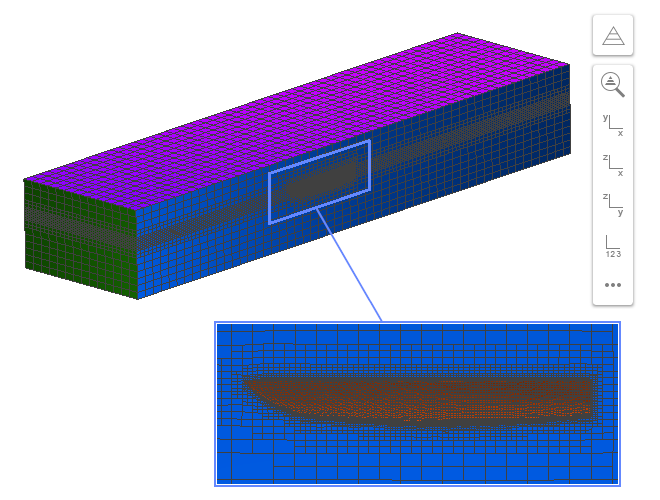## 18. Select Solver - Inter

To analyze water behaviour we will use Inter (interFoam) solver. This solver is designed to model two-phase flow with interface capturing capabilities.

1. Go to SETUP panel
2. Select filter
3. Select Multiphase model filter
4. Pick Inter (interFoam) solver from the list
5. solver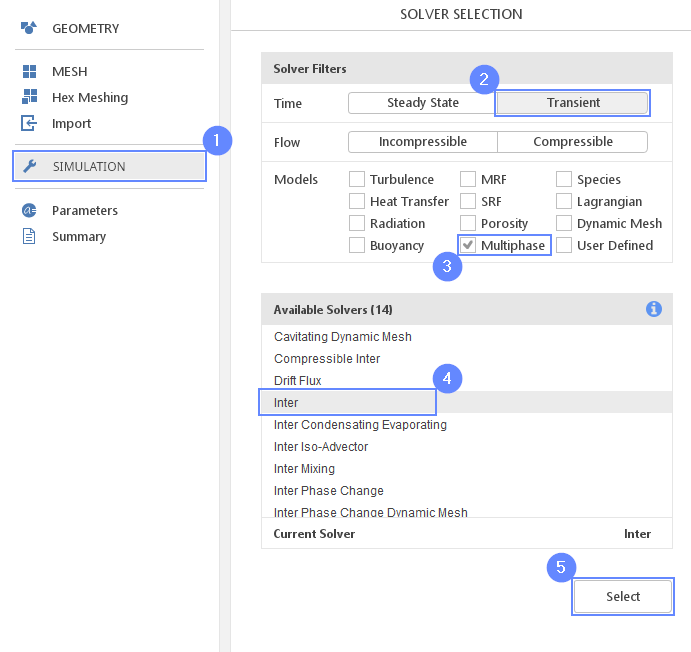## 19. Dynamic Mesh (I)

The dynamic mesh can be used for a simulation where the shape of the domain is changing. In our case, we will use dynamic mesh capabilities paired with six degrees of freedom (6 DOF) solver.

The 6DOF solver will predict the trajectory of a moving body using the aero or hydrodynamic forces and inertial properties assigned to a given boundary. During the analysis, the mesh will adjust to the new position of the body by moving nodes in the deformation region defined by the distance from the body.

1. Go to Dynamic Mesh panel
2. Choose Dynamic Mesh Type
3. Select the Boat geometry
4. Set the Inner Distance [m] to 0.2 for the deformation region
5. Set the boat mass properties
Mass $${\sf [kg]}$$120
Center of Mass $${\sf [m]}$$-1.10-0.1385
Mom. Of Inertia $${\sf [kg m^2]}$$100500100
6. Set the Relaxation to 1
 We use only half of the mass because of model symmetry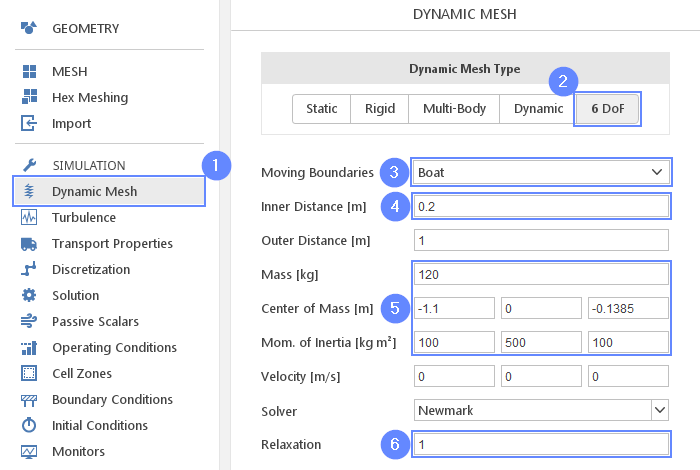## 20. Dynamic Mesh (II)

It is possible to constrain boundary motion. We will allow the boat to move only in the Z-axis direction and rotate about Y-axis. We will also enable damping to improve the stability of the calculation.

1. 2 Set the constraints accordingly
Translation Constraint Line
Rotation Constraint Axis
Axis $${\sf [-]}$$010
2. 3 Set the restraints accordingly
Linear Restraint Damper
Damping $${\sf [Ns/m]}$$30
Angular Restraint Damper
Damping $${\sf [Nms/m]}$$30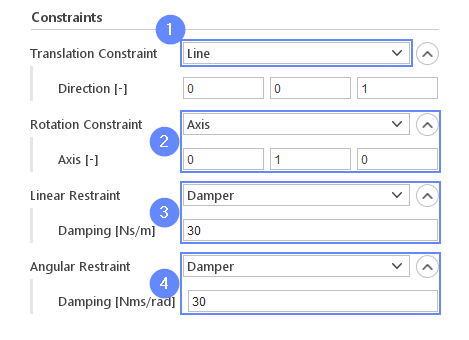## 21. Turbulence

For the purpose of this tutorial, we will model the turbulence phenomenon using the k-ω SST model.

1. Go to Turbulence panel
2. Select RANS turbulence formulation
3. Select $$k {-} \omega \; SST$$ model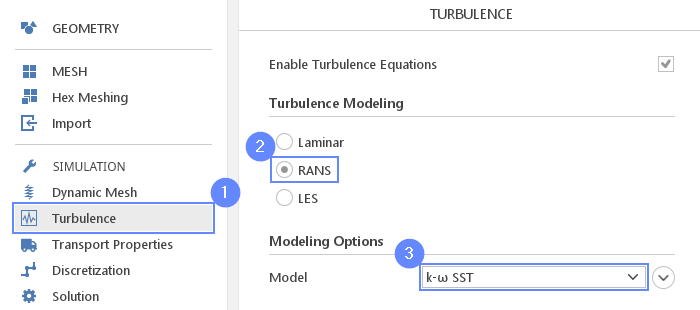## 22. Transport Properties - Water

In order to define water and air, we need to go to the transport properties panel, and use predefined fluids properties from the material database.

1. Go to Transport Properties panel
2. Change phase name from phase1 to water
3. Open
4. Pick up water from the list
5. Click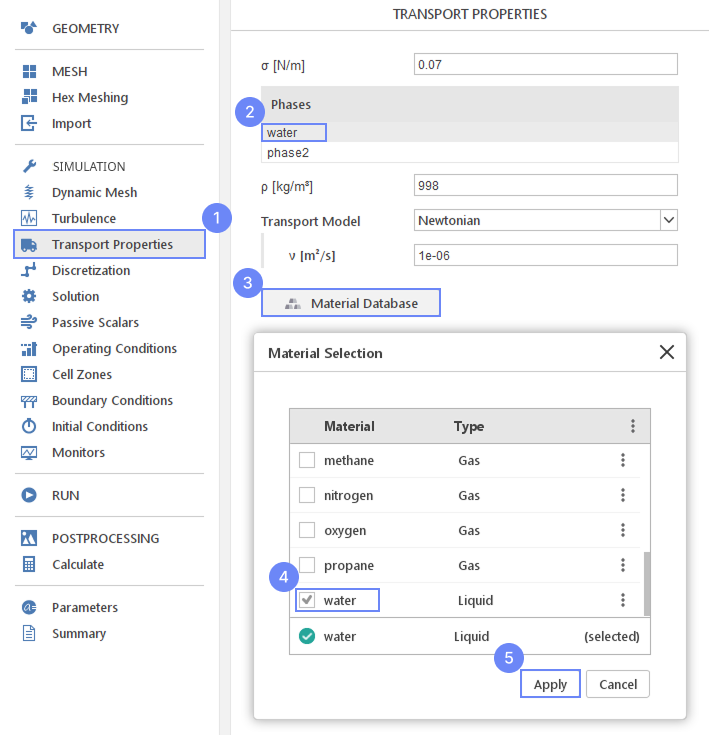## 23. Transport Properties - Air

Repeat previous step for phase2 using air properties.

To assign material to the domain, the phase fraction parameter $$\alpha_{phase}$$ is used. The parameter determines the proportion of each fluid in the domain.

The value of phase fraction varies in range from 0 to 1 where $$\alpha_{phase 1}=1$$ means that the whole domain is filled only with the phase1, while $$\alpha_{phase 1}=0$$ means that the domain is filled with the second phase.

We will assign $$\alpha_{phase}$$ parameter in the later steps.

1. Change phase name from phase2 to air
2. Open
3. Pick up air from the list
4. Click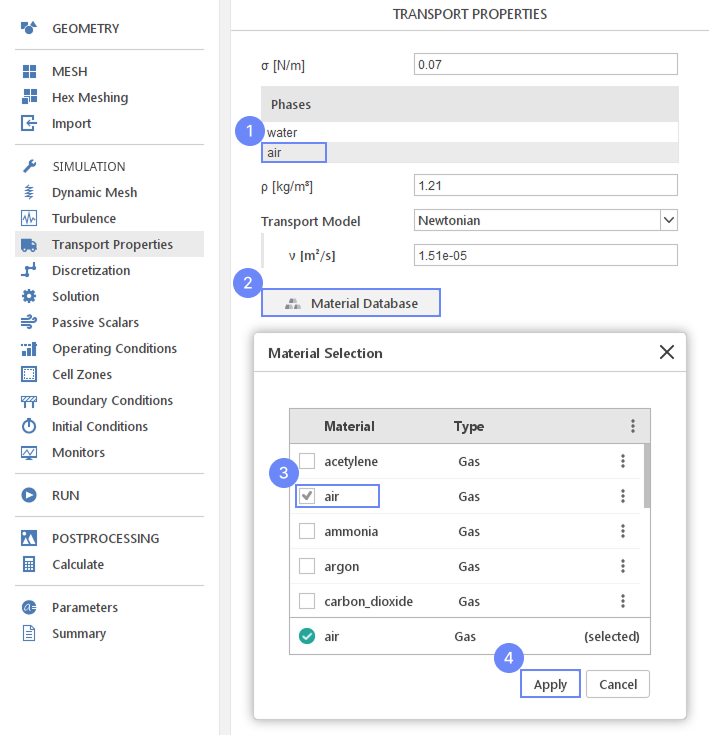## 24. Solution - PIMPLE

For the purpose of the simulation we will change PIMPLE algorithm parameters to increase stability:

1. Go to Solution panel
2. Switch to the PIMPLE tab
3. Increase Outer Correctors to 3
4. Increase Non-Orthogonal Correctors to 2
5. Uncheck Momentum Predictor for stability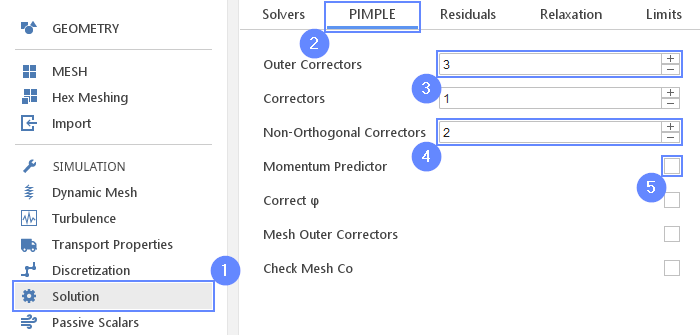## 25. Simulation Parameters

The velocity magnitude will be used in multiple simulation settings. It is handy to parameterize velocity value to be easily accessible in the future.

1. Go to Parameters panel
2. Define new parameter
NameU
Formula2
3. Press Enter or button
4. The newly created parameter will be shown in the parameters list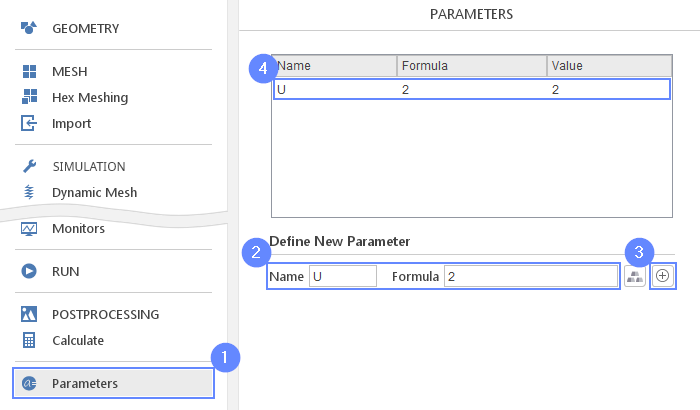## 26. Boundary Conditions - Bottom

In our simulation, the frame of reference will be associated with the boat and therefore we will simulate a fluid flow around the stationary boat. In order to properly represent ground in the boat frame of reference, we will enforce velocity at the bottom mesh boundary.

1. Go to Boundary Conditions panel
2. Select the bottom boundary
3. Change the Type of velocity to the Fixed Value
4. Set the Value $${\sf [m/s]}$$U00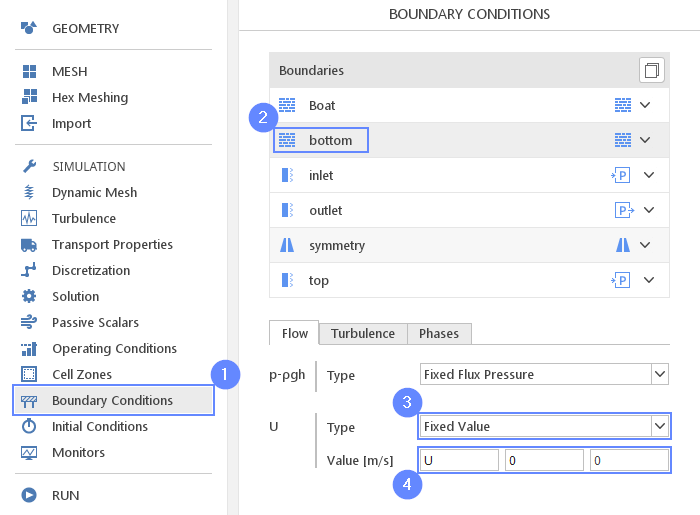## 27. Boundary Conditions - Inlet (Flow)

To model the inlet to the domain, we will assign the Inlet Velocity character and use the value of the "U" parameter as the inlet velocity value.

1. Select the inlet boundary
2. Set the character
3. Set the velocity Reference Value [m/s] to U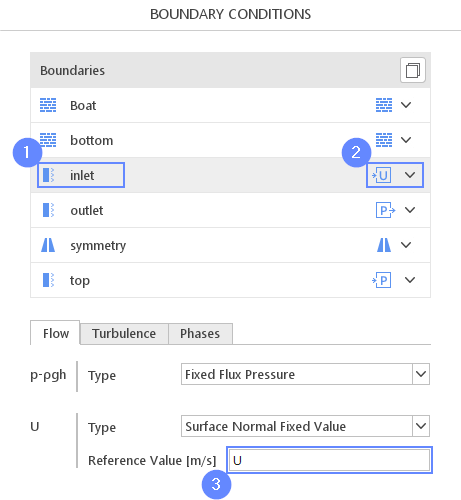## 28. Boundary Conditions - Inlet (Phases)

In addition to setting the flow conditions, selecting the inlet phase is also necessary.

Initially, we will specify pure air as the inlet phase. The water phase at the inlet will be patched later on by the water box geometry. Patching operation will modify the value parameter in a certain region, and effectively we will get an inlet of two phases from a single boundary.

1. Switch tab to Phases
2. Set the type to Fixed Value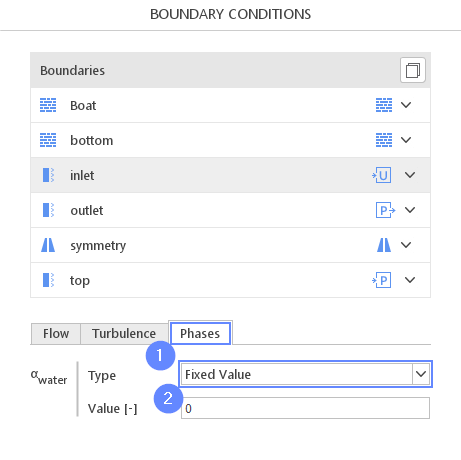## 29. Boundary Conditions - Outlet (Flow)

For the outflow boundary we will use Outlet Phase Mean Velocity condition. This boundary condition adjusts the velocity for the given phase to achieve the specified mean velocity.

1. Select the oulet boundary
2. Set the Outflow character
3. Switch tab to Flow
4. Set the velocity type and mean value accordingly
U Type Outlet Phase Mean Velocity
U Umean $${\sf [m/s]}$$U
 After you change flow boundary condition, the character will switch form Outflow to Custom. Do not change it back to Outflow afterwards.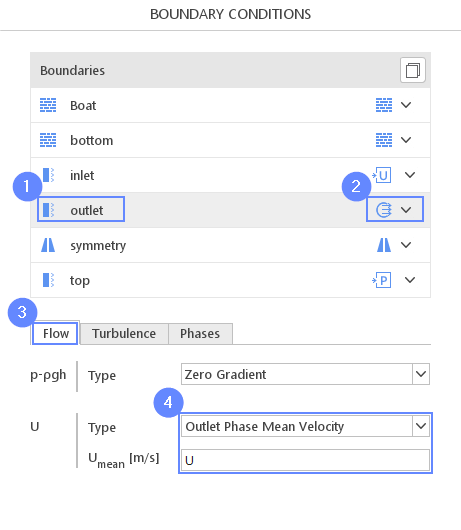## 30. Boundary Conditions - Outlet (Phases)

1. Switch tab to Phases
2. 3 Set the $$\alpha_{water}$$ parameters accordingly
$$\alpha_{water}$$ Type Variable Height
$$\alpha_{water}$$ Upper Bound $${\sf [-]}$$1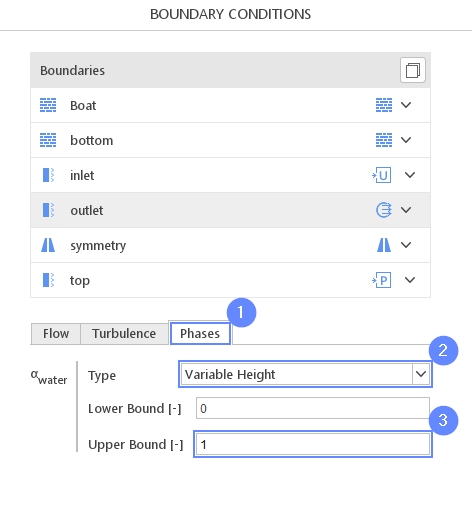## 31. Initial Conditions - Basic

Before we start simulation, we need to define the initial state.

We will use parameter U to initiate constant velocity in the domain. The domain will initially be filled entirely with air, as indicated by a phase fraction $$\alpha_{air}=0$$.

1. Go to Initial Conditions panel
2. Set the velocity to U00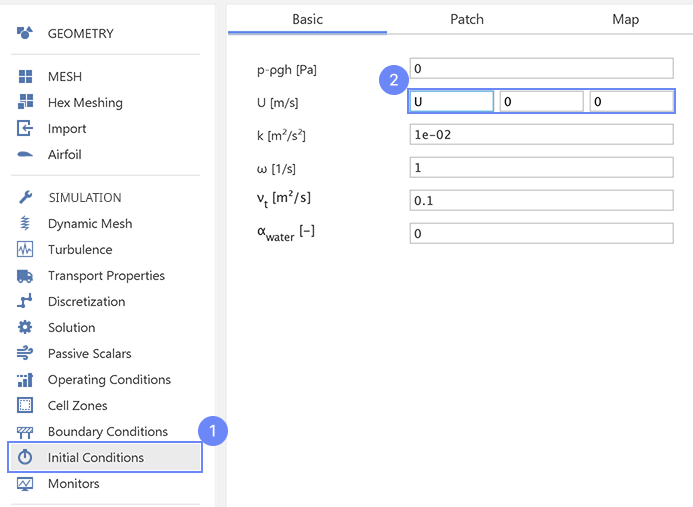## 32. Initial Conditions - Patch

Using the water geometry, we will overwrite the phase fraction value inside it. We will set the $$\alpha_{water}$$ to 1 to fill the patched geometry with the water.

1. Switch to Patch tab
2. Enable initialization on water geometry
3. Expand list
4. Select $$\alpha_{water}$$ fraction for initialization
5. Set initial value of $$\alpha_{water}$$ to 1
6. Apply patch for Both cells and faces. This will override values at boundaries as well.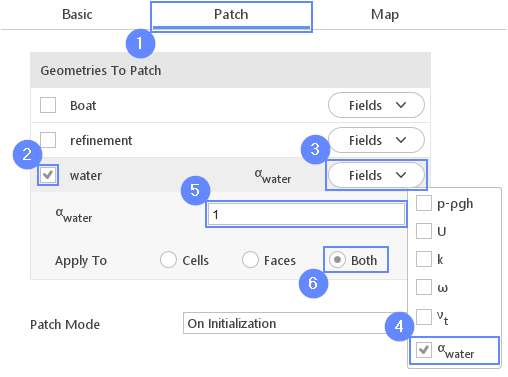## 33. Monitors - Sampling

During calculation, we can observe intermediate results on a section plane.

To add sampling data on a plane we need to define plane properties and also select fields that will be sampled. Note that runtime post-processing can only be defined before starting calculations and can not be changed after the simulation has started.

1. Go to Monitors panel
2. Switch to Sampling tab
3. Select
4. Set the slice normal vector align y-axis
Normal $${\sf [-]}$$010
5. Expand list
6. Check the velocity U
7. Check the water phase $$\alpha_{water}$$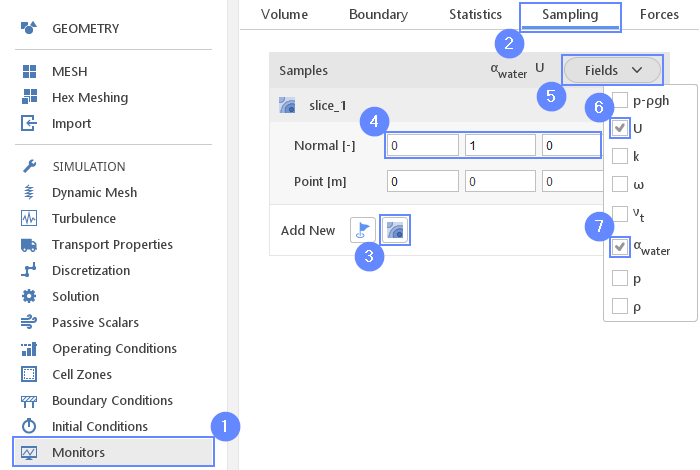## 34. Monitors - Forces

Additionally, we will observe forces acting on the boat boundary.

1. Switch to Forces tab
2. Expand Monitored Boundaries list and check Boat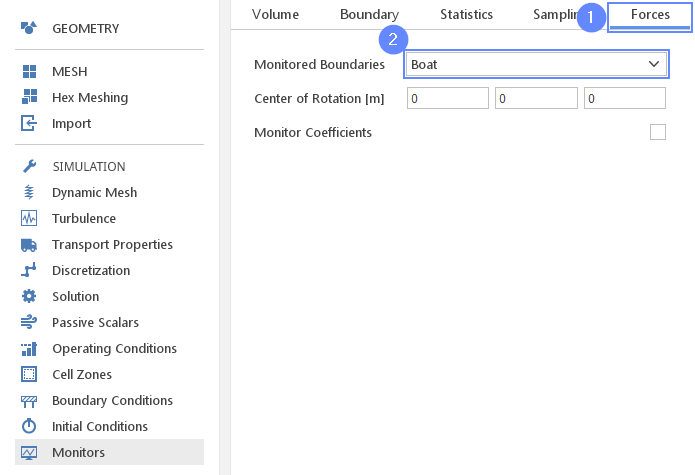## 35. Run - Time Control

For any simulation, it is very convenient to let the solver automatically determine the proper time step value. To use this option we need to define time step constraints by providing the initial time step(adjusted by the solver during computations) and maximal time step value. Rest of the parameters we can leave the default.

1. Go to RUN panel
2. Set the Simulation Time [s] to 10
3. Change Time Stepping to Automatic
4. Set initial and maximum timesteps (solver will start computation with the initial value and adjust it in the next iterations not exceeding the maximum value)
Initial $$\Delta t$$ $${\sf [s]}$$1e-04
Max $$\Delta t$$ $${\sf [s]}$$1e-02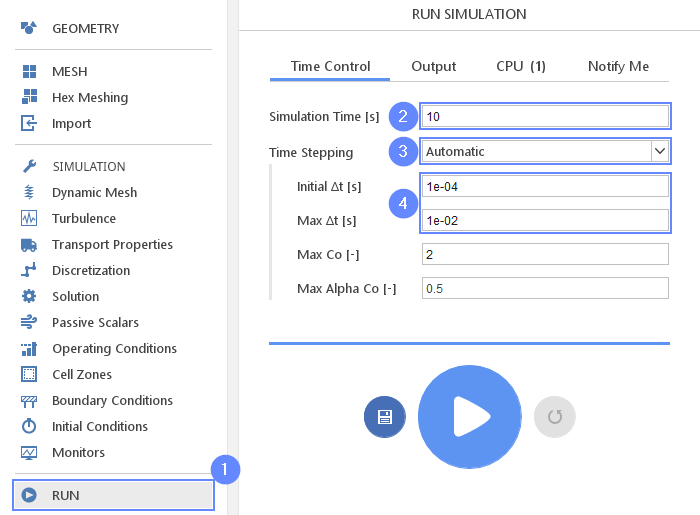## 36. Run - Output

We can control how often results should be saved on the hard drive. Only this data will be available for postprocessing.

1. Switch to Output tab
2. Set the Write Control Interval [s] to 0.05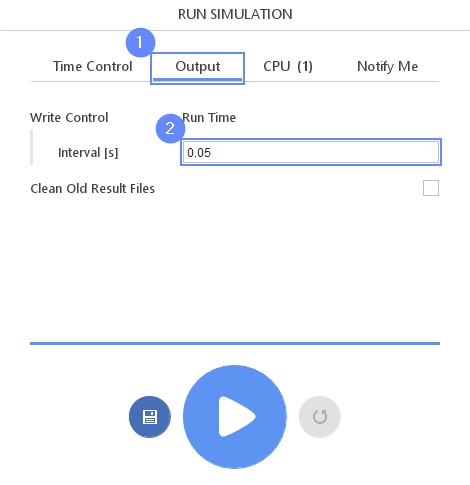## 37. Run - CPU

The calculation of this simulation is very time-consuming, due to the dynamic mesh and 2 phase model. To speed up the calculation process we recommend using at least 8 cores for this tutorial.

If you are using SimFlow free version (which allows only for 2 CPUs) you can use the contact form to Request 30-day Trial

Estimated computation time for 2 CPUs is over 5 hours.

1. Switch to CPU tab
2. Use parallel mode
3. Increase the Number of processors
4. Click button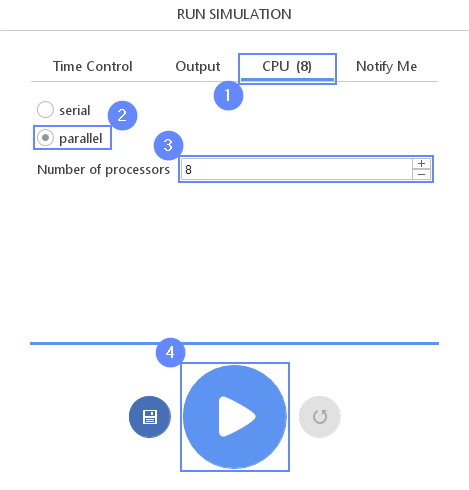## 38. Postprocessing - ParaView

Once the computations have been completed, we can perform advanced visualization of the results using ParaView.

1. Go to POSTPROCESSING panel
2. Click on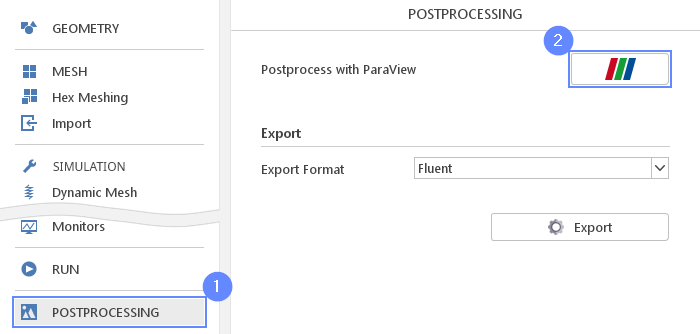## 39. ParaView - Load Results

Load the results into the program.

1. Turn on
2. Uncheck option Decompose polyhedra
3. Click to load results into ParaView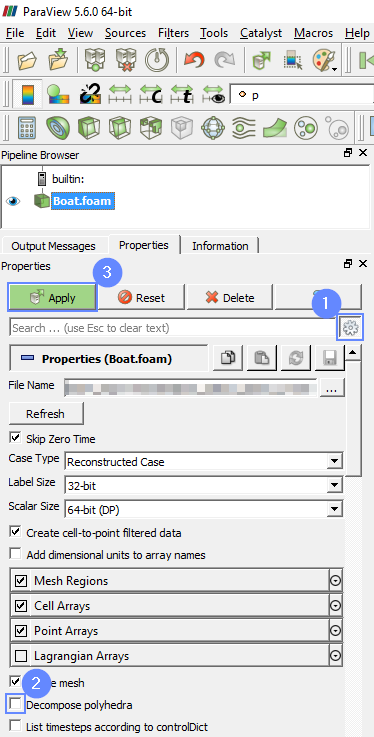## 40. ParaView - Dynamic mesh

We will look at the dynamic mesh displacement.

As we can see, the boat can move along Z-axis and rotate around Y-axis. The mesh at a distance of 0.2 m from the boat is rigid and moves together with a boat. The mesh at the distance from 0.2 m to 1 m from the boat is able to move. Further than 1 m from the boat, the mesh is non-deformable.

1. Select Surface with Edges from the representation toolbar list
2. Select pointDisplacement from the variable list
3. Click
4. Play with an animation buttons to track the results of analysis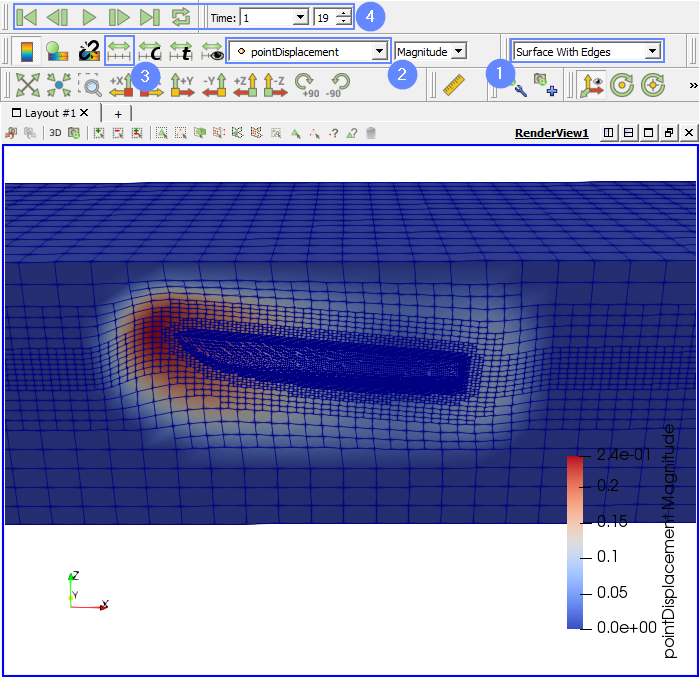## 41. ParaView - Reflect the results (I)

During the simulation, only one half of the fluid domain was used. However, during the post-processing stage, we can replicate the second half of the domain.

To produce a visualization that is symmetrical, we will reflect the results across the plane of symmetry.

1. Select the case Boat.foam
2. Extend the list of Filters from the top menu
3. Go to Alphabetical
4. Select Reflect from the list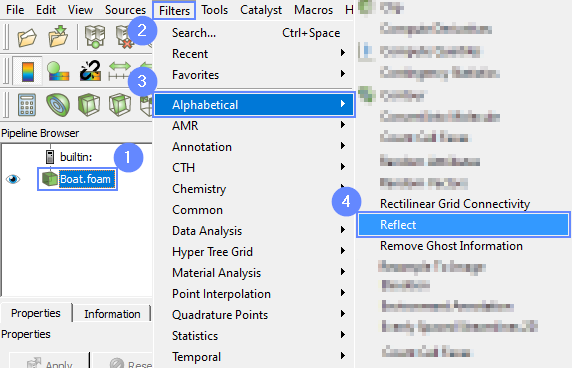## 42. ParaView - Reflect the results (II)

1. Choose the plane of the reflection Y min
2. Click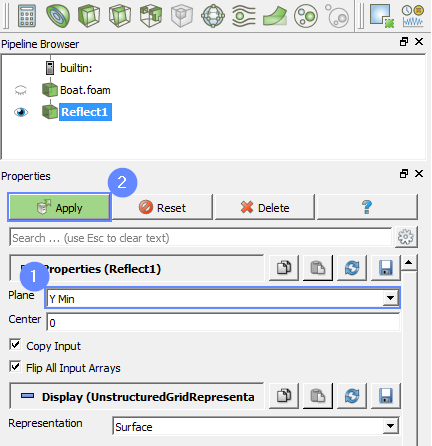## 43. ParaView - Water Surface

We will focus on the water-air interface behaviour and observe the velocity map.

2. Change the clip type to Scalar
3. Select alpha.water from the scalar list
4. Click
5. Make sure Invert option is unchecked
6. Click
7. Select the velocity U from the list
8. Click on
9. Set the range from 0 to 3
10. Confirm by clicking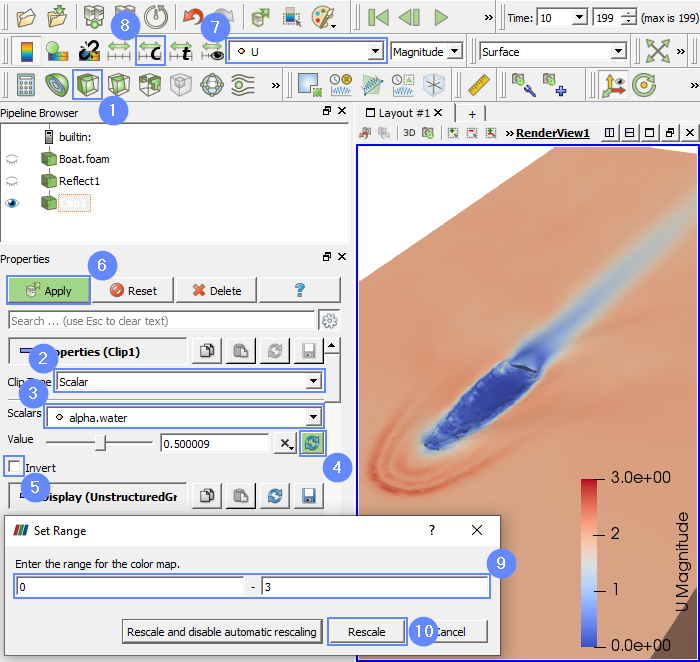## 44. ParaView - Boat Geometry (I)

To display the boat geometry, we will read the result file once again and load only the boat boundary.

2. Choose the Boat.foam file from case folder
3. Press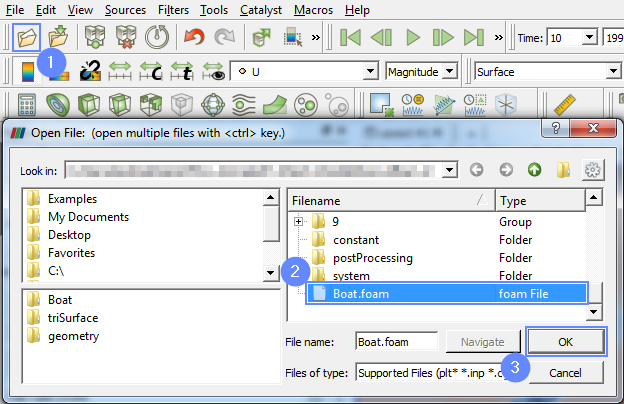## 45. ParaView - Boat Geometry (II)

1. Uncheck internalMesh from the Mesh Regions
2. Check the Boat
3. Press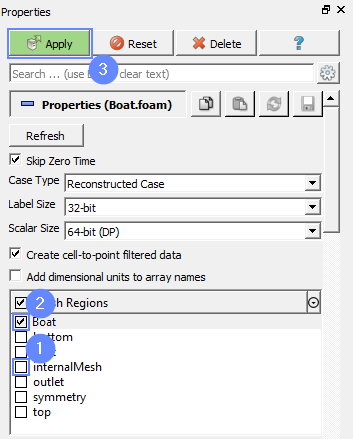## 46. ParaView - Boat Geometry (III)

Similarly as before, we will reflect the boat geometry.

1. Select the new case name Boat.foam, go to Filters, extend Alphabetical and select Reflect from the list
2. Choose the plane of reflection Y min
3. Click
4. Select the Solid Color from the list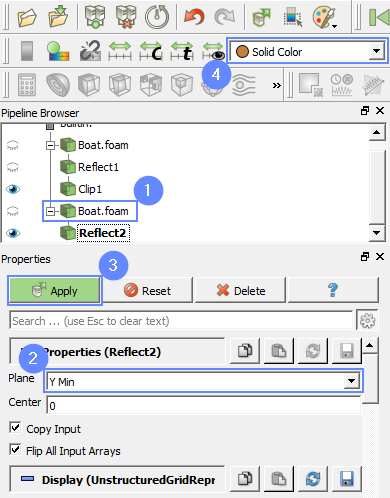## 47. ParaView - Results

1. Play with animation buttons to investigate the time history of the flow.